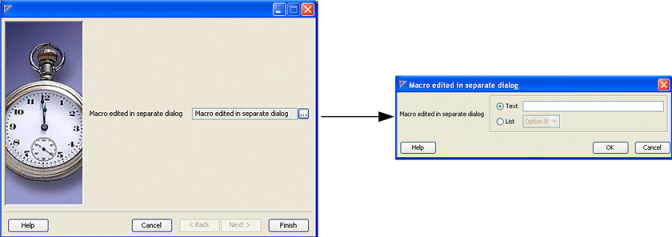Application Developer's Guide
Using the Rule Wizard framework : Using editors in separate dialogs

# Using editors in separate dialogs

Whenever you want to save space on a wizard page (because many other editors show), use a proxy editor to display the macro value but not all the GUI components required for editing. Alternatively, you can do the editing in a separate window, by modifying the fourth example, as illustrated in the following example. Use the named editor, aggregate_editor, in order to pass it as a parameter (editorName) to the proxy editor implemented by the com.savvion.rulewizard.gui.FieldEditor. The macro, editor definition, and code definition portion remain unchanged. The proxy editor contains its own label and also a columns parameter.
<?xml version="1.0" encoding="UTF-8"?>
<!DOCTYPE template SYSTEM "resources/ruleTemplate.dtd" >
<template name="ex6">
<editor name="aggregate_editor" macro="aggregate">
<editor macro="text" label="Text">
<parameter name="columns" value="30"/>
<label-constraints fill="HORIZONTAL" insets="2,2,2,2" weightx="1"
gridx="0" gridy="0"/>
<constraints fill="NONE" insets="2,2,2,2" weightx="2" gridx="1"
gridy="0" anchor="WEST"/>
</editor>
<editor macro="list" label="List">
<label-constraints fill="HORIZONTAL" insets="2,2,2,2" weightx="1"
gridx="0" gridy="1"/>
<constraints fill="NONE" insets="2,2,2,2" weightx="2" gridx="1"
gridy="1" anchor="WEST"/>
</editor>
</editor>
<screen number="10">
<editor macro="aggregate" class="com.savvion.rulewizard.gui.FieldEditor"
label="Macro edited in separate dialog">
<parameter name="editorName" value="aggregate_editor"/>
<parameter name="columns" value="20"/>
</editor>
</screen>
<macro name="aggregate" type="composite">
<vector-property name="groupComponents">
<vector-elem>text</vector-elem>
<vector-elem>list</vector-elem>
</vector-property>
The template generates the following screen (left image, Figure 28). Click the ellipsis button to open the dialog box (right image, Figure 28).
Figure 28. Rule wizard example 6